# RD Sharma Solutions For Class 12 Maths Exercise 19.14 Chapter 19 Indefinite Integrals

RD Sharma Solutions for Class 12 Maths Exercise 19.14 Chapter 19 Indefinite Integrals is provided here. Some special integrals along with proof are dealt with in this exercise. By using these results, the given integrals should be solved. For students who are finding it difficult to understand and solve problems, what you all need is RD Sharma Solutions for Class 12.

Experts at BYJU’S have created these answers, keeping in mind the concept level of the students to meet their needs. The detailed solutions will help students secure good marks in the subject. The PDF of RD Sharma Solutions for Class 12 Maths Chapter 19 Exercise 19.14 is provided here. Students can refer and download from the below-provided links.

## Download the PDF of RD Sharma Solutions For Class 12 Chapter 19 – Indefinite Integrals Exercise 19.14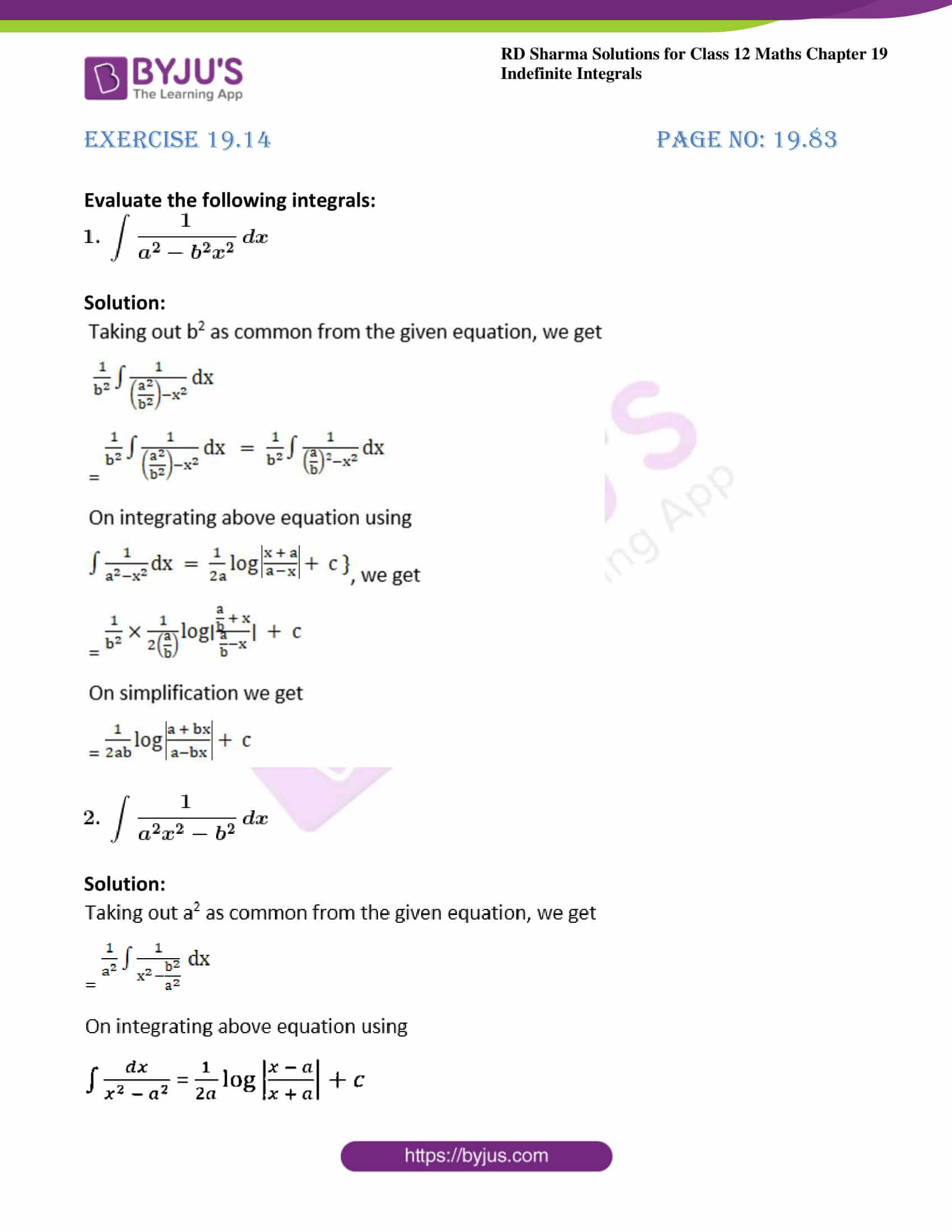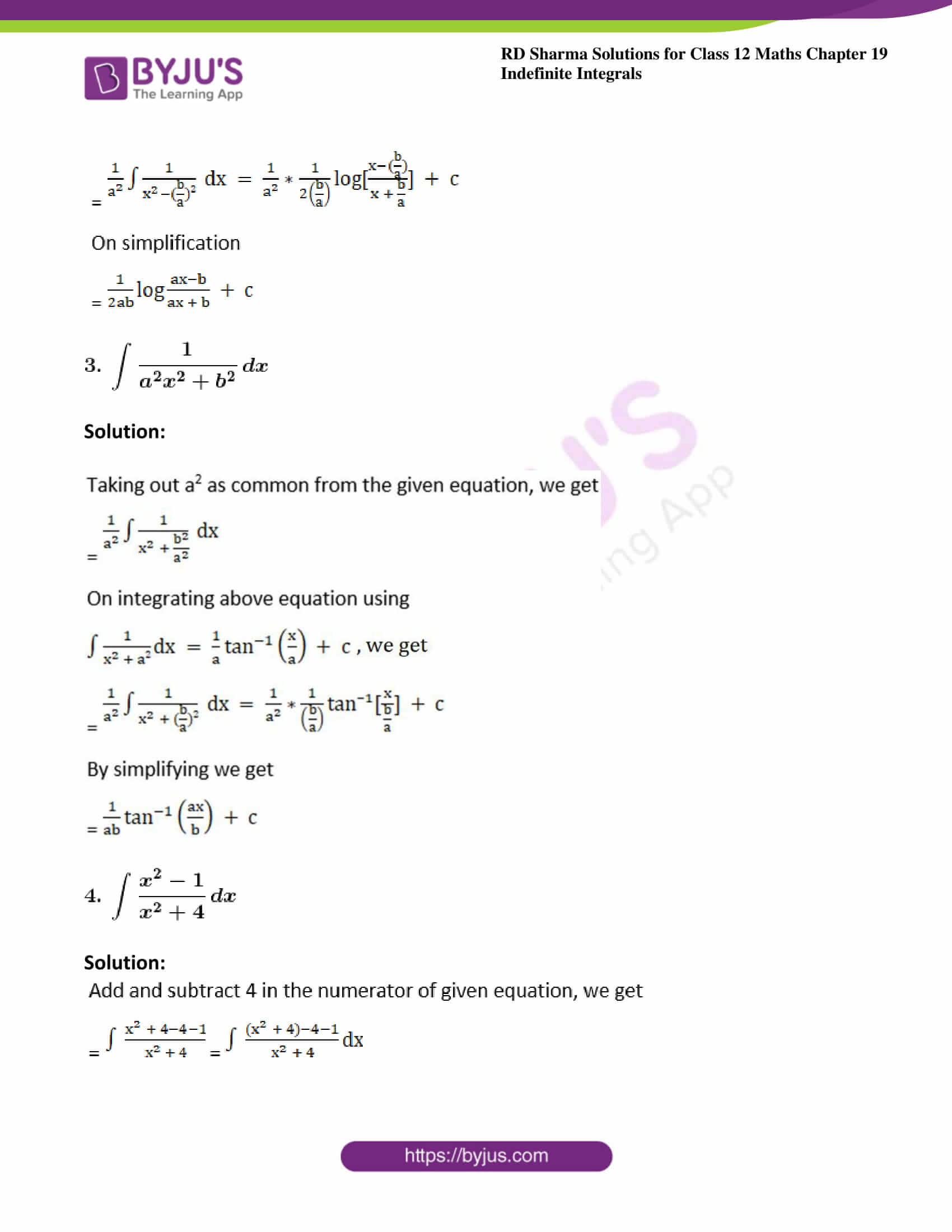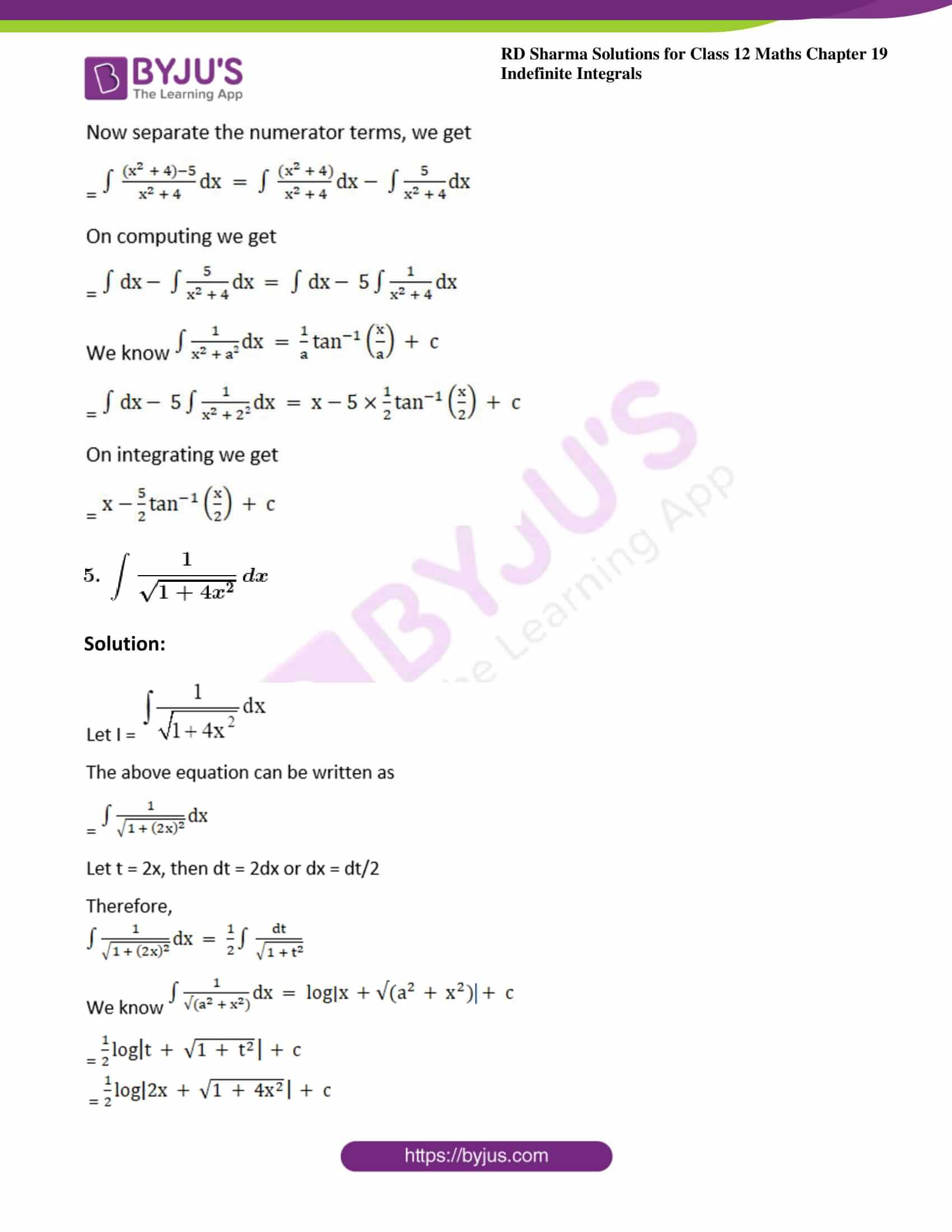### Exercise 19.14 Page No: 19.83

Evaluate the following integrals: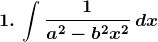Solution: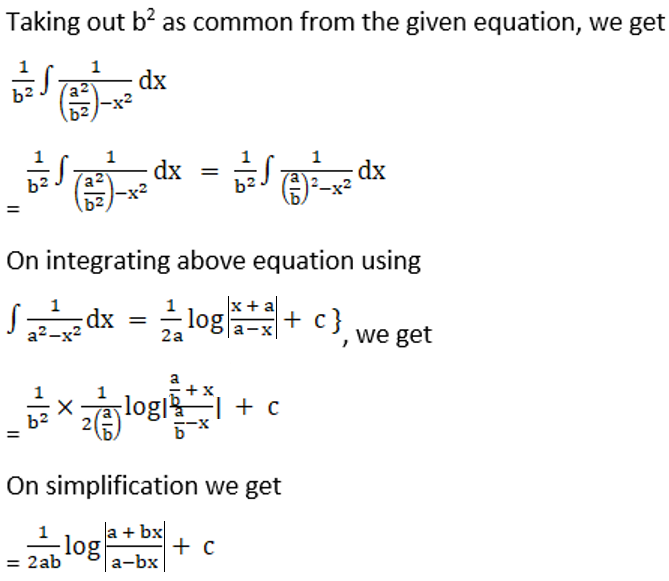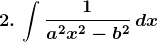Solution: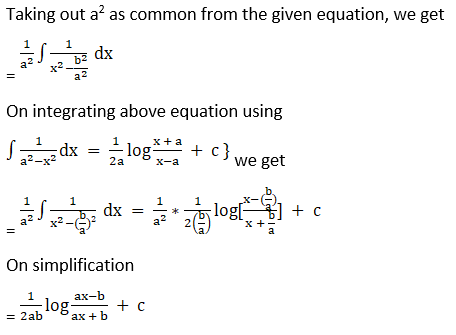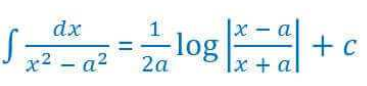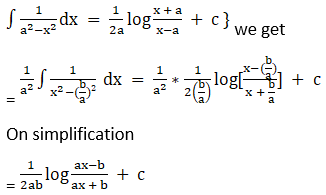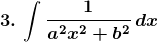Solution: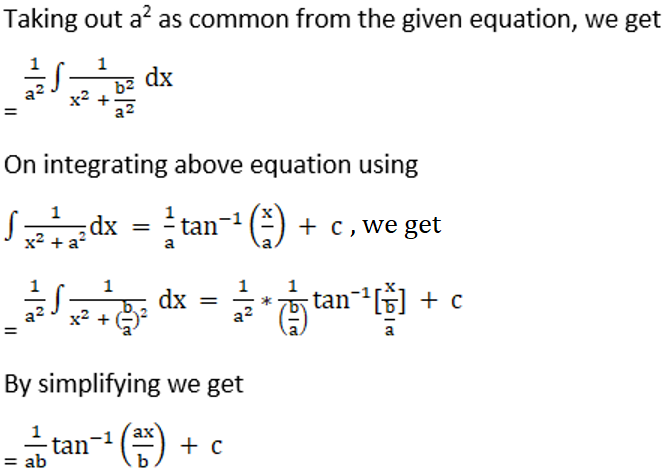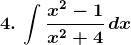Solution: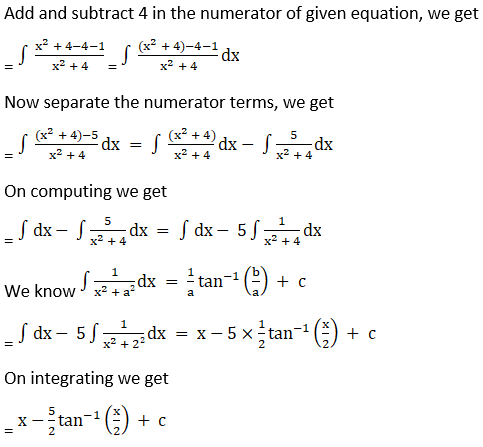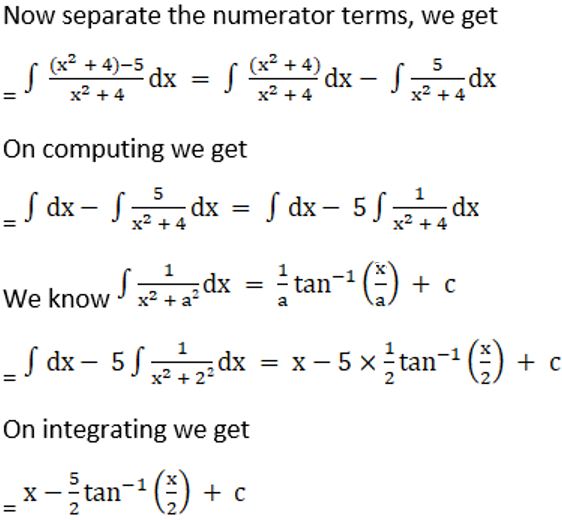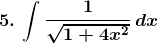Solution: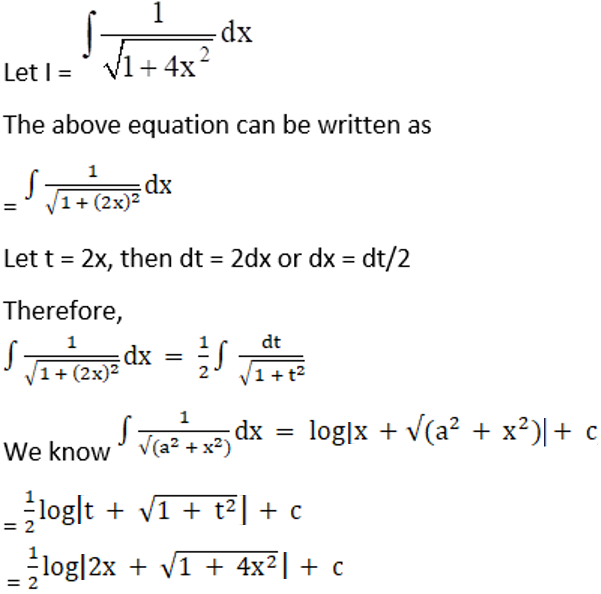### Access other exercises of RD Sharma Solutions For Class 12 Chapter 19 – Indefinite Integrals

Exercise 19.1 Solutions

Exercise 19.2 Solutions

Exercise 19.3 Solutions

Exercise 19.4 Solutions

Exercise 19.5 Solutions

Exercise 19.6 Solutions

Exercise 19.7 Solutions

Exercise 19.8 Solutions

Exercise 19.9 Solutions

Exercise 19.10 Solutions

Exercise 19.11 Solutions

Exercise 19.12 Solutions

Exercise 19.13 Solutions

Exercise 19.15 Solutions

Exercise 19.16 Solutions

Exercise 19.17 Solutions

Exercise 19.18 Solutions

Exercise 19.19 Solutions

Exercise 19.20 Solutions

Exercise 19.21 Solutions

Exercise 19.22 Solutions

Exercise 19.23 Solutions

Exercise 19.24 Solutions

Exercise 19.25 Solutions

Exercise 19.26 Solutions

Exercise 19.27 Solutions

Exercise 19.28 Solutions

Exercise 19.29 Solutions

Exercise 19.30 Solutions

Exercise 19.31 Solutions

Exercise 19.32 Solutions